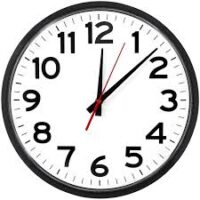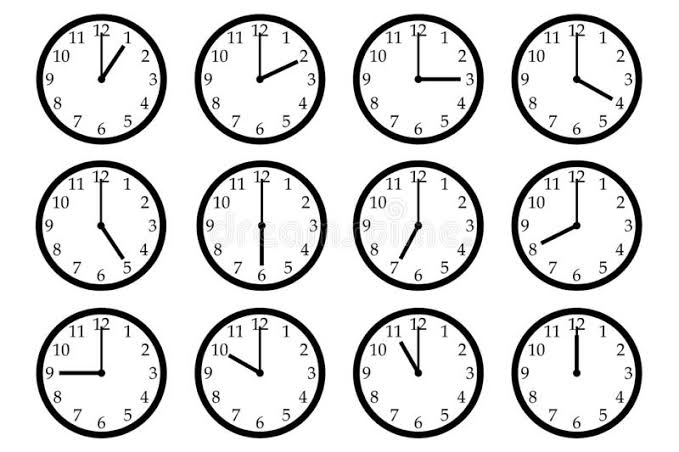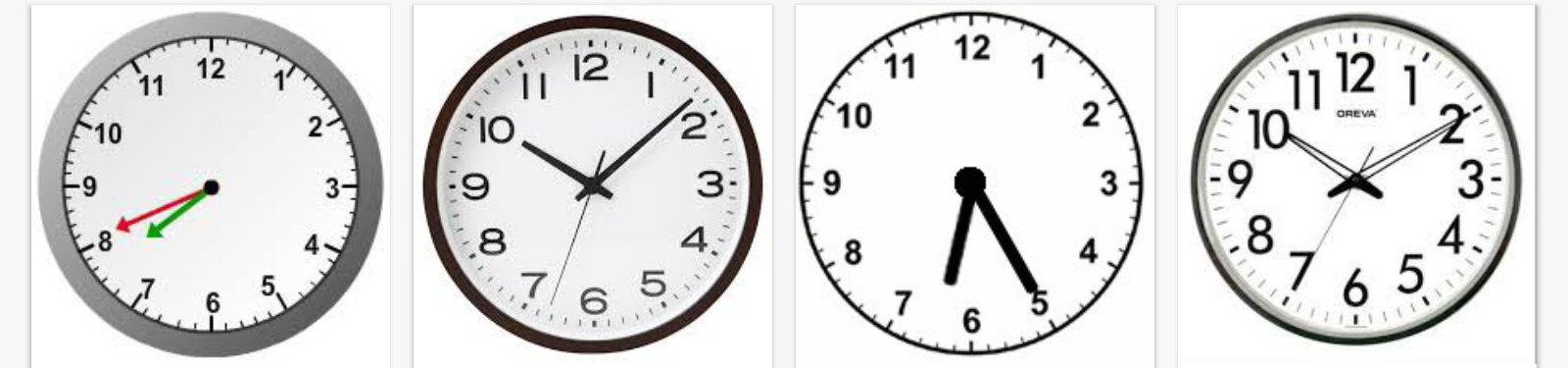# Time, Distance and Average Speed | Word Problems (Real Life Application) Primary 6 (Basic 6) Term 3 Week 4 Mathematics

MATHEMATICS

THIRD TERM

WEEK 4

PRIMARY 6

THEME –

PREVIOUS LESSON – Polygons – 2 Dimensional Shapes Not Exceeding Octagon | Lines of Symmetry Primary 6 (Basic 6) Term 3 Week 4 Mathematics

#### LEARNING AREA

1. Introduction

2. Time and Distance

3. Average Speed

4. Real Life Application (Word Problems)

5. Lesson Evaluation and Weekly Assessment (Test)

#### LEARNING OBJECTIVES

By the end of the lesson, most pupils should have attained the following objectives –

1 tell the time on a 12 and 24-hour clock.

3. calculate the time, distance and average speed.

4. solve quantitative aptitude problems on time,distance and speed.

#### ENTRY BEHAVIOUR

The pupils can appreciate the importance of time in everyday life

#### INSTRUCTIONAL MATERIALS

The teacher will teach the lesson with the aid of clock face, stop watch, railway timetable, bus timetable, air timetable.

#### METHOD OF TEACHING

Choose a suitable and appropriate methods for the lessons.

Note – Irrespective of choosing methods of teaching, always introduce an activities that will arouse pupil’s interest or lead them to the lessons.

### REFERENCE MATERIALS

1. Scheme of Work

2. 9 – Years Basic Education Curriculum

3. Course Book

4. All Relevant Material

5. Online Information

### LESSON 1 – INTRODUCTION

ACTIVITY 1 – TIME (5 minutes)

A clock is used to tell time in hour and minute(s).

The face of the clock shows 12 hour clock times with two or three hands.The long and thick hand on the clock face is called the minute hand.

The short and thick hand on the clock face is called the hour hand.

While the long and thin hand on the clock face is called the second hand.

ACTIVITY 2 – USES OF CLOCK AND TIME (5 minutes)

Clock is used to tell time while

• Time makes us plan our daily activities well.
• Time is used as a reminder.
• Time is used to set priority.
• Time is money.

ACTIVITY 3 – QUICK EXERCISE (10 minutes)

1. Tell the time for the following:

• Assembly
• Short break
• Long break
• Closing time

2. If 60 seconds = 1 minutes, 60 minutes = 1 hour and 3 600 seconds = 1 hour.

Copy and complete,

• 2 minutes = _____ seconds
• 3 hours = _____ minutes
• 3 hours = _____ seconds
• 600 seconds = _____ minutes
• 600 seconds = _____ hours

Hint – when changing seconds to minutes, divide by 60. Changing minutes to seconds, multiply by 60. Minutes to hour, divide by 60 while hour to minutes, multiply 60.

ACTIVITY 4 – TELLING TIME (5 minutes)

Teacher organizes pupils in groups or pairs depending on the size of the class and available clock charts to tell the following time –Whenever the long hand is on 12 and the short hand is on any number, the time is that number.

For example – 1 o’clock, 2 o’clock, etc. as shown in the above clocks.

ACTIVITY 5 – CLASS EXERCISE (15 minutes)

If 24 hours make one day, how hours are there in

1. 2 days

2. A week

How many weeks are in

3. A month

4. 6 months

How many months are in

5.1½ years

6. 5 years

### LESSON 2 – AVERAGE SPEED

ACTIVITY 1 – READING TIME IN 12 AND 24 HOURS (5 minutes)

Using the existing groups or pairs, teacher asks pupils to tell the time on the clocks and also, guides them to tell the equivalent time in 24 hour clock.The 12 hour clock runs from 1am to 12 noon and then from 1pm to 12 am midnight while the 24 hour clock uses the numbers that runs from 00:00 midnight to 23:59.

For example,

12 Hour Clock                                                  24 Hour Clock

• Clock 1 – 7:41 am                                                                07:41
• Clock 2 – 10:18 pm                                                              22:18
• Clock 3 – 6:25 pm                                                                18:25
• Clock 4 – 10:10 am                                                              10:10

Hint – When 24-hour clock times are written with four figures, the first two figures represent hours and the last two figures represent minutes past the hour.

ACTIVITY 2 – CLASS EXERCISE (5 minutes)

Write each time as a 24-hour time.

1. 3.25 p.m

2. 10.24 a.m

3. 11.13 p.m

Write each time as a 12-hour time. Use a.m or p.m.

4. 18.00

5. 19.50

6. 10.10

ACTIVITY 2 – DISTANCE (2 minutes)

Distance is the length of the space between two points.

For example, the distance between Lagos to Ibadan, etc.

Distance is most commonly measured in millimeters, centimeters, meters, and kilometers.

ACTIVITY 3 – AVERAGE SPEED (3 minutes)

The average speed is the total distance traveled by the object in a particular time interval.

The most common formula for average speed is distance traveled divided by time taken.

The basic unit of average speed is km/h or m/s.

That’s, Average Speed =

### Time Taken

ACTIVITY 4 – WORKING EXAMPLE 1

A car travels 3 900 km in 5 hours. What is its average speed?

SOLUTION

Given, total distance travelled is 3 900 km and the time taken is 5 hours.

Therefore,

3 900 km

____________

5 h

= 780 km/h

ACTIVITY 5 – WORKING EXAMPLE 2

AyanfeOluwa walked a distance of 120 metres in a minute. Find his walking speed in km/h.

SOLUTION

Given, total distance covered is 120 m and time taken is 1 minute.

Note, you are to find the average speed in km/h. Therefore, change the metres to kilometres and the minute to hour.

That’s,

120 x 1

____________ km/h

1000 x 60

= 1/500 or 0.002 km/h

ACTIVITY 3 – WORKING EXAMPLE 3

An aeroplane flies 100 m in 1 hour. Find the average speed in m/s.

SOLUTION

Total distance is 100 m and time taken is 1 hour (60 seconds).

Therefore, average speed =

100

____________ m/s

60

= 1.67 or 1 2/3 m/s

ACTIVITY 4 – CLASS EXERCISE

1. A car travelled 5 040 km in 6 hours. Find its average speed.

2. If it takes Treasure 3 hours to drive a distance of 192 km on a motorway, what is the average speed in km/h?

3. A bicycle travels a distance of 500 km in 20 hours. What is the average speed in km/hr?

4. Caleb can walk a distance of 150 m in 20 seconds. Find the average speed of Caleb in m/s.

5. Copy and complete the following:

Total Distance           Time Taken           Average Speed

100 m                                 5s

2530 km                            15h

155 m                                 1 h

732 km                               120s

### LESSON 3 – TIME AND DISTANCE

ACTIVITY 1 – INTRODUCTION

If Average Speed =

Time =

Distance =

### Speed x Time

ACTIVITY 2 – WORKING EXAMPLE 2

The average speed of a small car is 35 km/h. How long did it take for a journey of 140 km?

SOLUTION

Given, average speed = 35 km/h and distance = 140 km

Therefore, time =

140

___________

35

= 4 h

ACTIVITY 3 – WORKING EXAMPLE 3

A train travelled at average speed of 560 km/h. What is the distance covered in 15 minutes.

SOLUTION

Given, average speed = 560 km/h and time taken = 15 m.

Therefore, distance covered =

560 x 15

= 8 400 km.

ACTIVITY 4 – WORKING EXAMPLE 4

A car travelled in 5 km in 20 minutes.

1. Find the average speed.

2. What is the distance covered in 2½ hours?

SOLUTION

1. Given, distance = 5 km and time = 20 m (20/60)

Average speed =

5 ÷ 20/60 =

5 x 60³

___________

20¹

= 15 km/h

2. Average speed = 15 km/h and time = 2½ (5/2) h

Therefore, distance =

15 km/h x 5/2 h

= 75/2 km

= 37.5 km or 37 1/2 km

ACTIVITY 5 – WORKING EXERCISE

1. What is the distance covered by a truck traveling at an average speed of 120 km/h in 45 minutes?

2. A car is traveling at an average speed of 150 km/h, how long is it going to take to travel 180 km?

3. A car travels a 600 km long street in 5 minutes. What is its average speed in km/h?

4. The distance between two stations is 1080 km. A car takes 6 hours to cover this distance. Calculate the average speed of the car in km/h and m/s.

5. A man walks at 20 km/h for 4 hours and then at 26 km/h for 2 hour. Find his average speed.

### LESSON 4 – PROBLEMS SOLVING (REVISION)

Teacher organizes pupils in groups or pairs depending on the size of the class and guides the pupils to analyze and solve questions (most especially in the examples and exercises on time, distance and average)

### LESSON 5 – REVISION AND WEEKLY ASSESSMENT

As stated in performance objectives or lesson evaluation.

### PRESENTATION

To deliver the lesson, the teacher adopts the following steps:

1. To introduce the lesson, the teacher revises the previous lesson. Based on this, he/she asks the pupils some questions;

2. Teacher organizes the pupils in groups or pair depending on the size of the class and available instructional materials.

3. Teacher displays samples of clocks or wristwatches.

4. Teacher asks pupils to discuss and state the importance of clock or wristwatch.

5. Teacher uses the instructional materials and pupil’s responses to introduce the lesson and guides pupils to identify the relationship between seconds, minutes and hours.

Pupil’s Activities – Pay attention to the lesson introduction to understand the concept of the lesson and solve problems on time.

6. Teacher leads a review on the relationship between metres and kilometres.

Pupil’s Activities – Participate actively to the lesson reviews on metres and kilometres.

7. Teacher uses the review to introduce average speed and guides pupils as groups or pairs to analyze questions on average speed and solve them.

Pupil’s Activities – Solve problems on average speed.

8. Teacher uses the average speed to analyze and discuss the relationship between time, distance and speed.

9. Teacher leads the class to solve more problems on time, distance and average speed.

Pupil’s Activities – Solve more problems on time, distance and average speed.

10. Teacher summarizes the lesson on the board with appropriate evaluation.

Pupil’s Activities – Participate actively in the summary of the lesson and write as instructed.

### CONCLUSION

To conclude the lesson for the week, the teacher revises the entire lesson and links it to the following week’s lesson.

### LESSON EVALUATION

As stated in working examples and exercises.

THANKS FOR USING CLASSROOMNOTE!!!